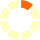# New York State Next Generation Learning Standards: 5.NF.5.b

## Explain why multiplying a given number by a fraction greater than 1 results in a product greater than the given number (recognizing multiplication by whole numbers greater than 1 as a familiar case). Explain why multiplying a given number by a fraction less than 1 results in a product smaller than the given number. Relate the principle of fraction equivalence a/b = a/b × n/n to the effect of multiplying a/b by 1.e.g.,Explain why 4 × 3/2 is greater than 4.Explain why 4 × 1/2 is less than 4.1/3 is equivalent to 2/6 because 1/3 × 2/2 = 2/6. .